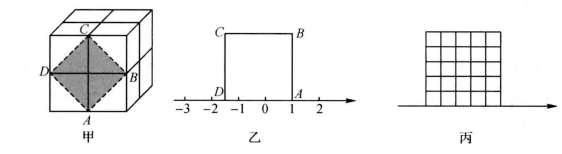(1)求这个魔方的棱长; (用代数式表示)
(2) 当魔方体积 $V=64 \mathrm{~cm}^3$ 时,
(1)图甲中阴影部分是一个正方形 $A B C D$, 求出阴影部分的面积;
(2)把正方形 $A B C D$ 放置在数轴上, 如图乙所示, 使得点 $A$ 与数 1 重合, 则 $D$ 在数 轴上表示的数为
(3) 在图丙的 $5 \times 5$ 方格中, 画一个面积为 13 的格点正方形 (四个顶点都在方格的顶 点上); 并把图中的数轴补充完整, 然后用圆规在数轴上表示实数 $\sqrt{13}$.①点击 首页查看更多试卷和试题 , 点击查看 本题所在试卷